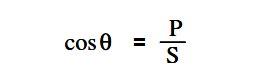# Power Factor

Power factor (pf) is the ratio between true power and apparent power.

True power is the power consumed by an AC circuit, and reactive power is the power that is stored in an AC circuit.

Cosθ is called the power factor (pf) of an AC circuit. It is the ratio of true power to apparent power, where θ is the phase angle between the applied voltage and current sine waves and also between P and S on a power triangle (Figure1).

The below Equation is a mathematical representation of power factor.where

cosθ = power factor (pf)
P = true power (watts)
S = apparent power (VA)

Power factor also determines what part of the apparent power is real power. It can vary from 1, when the phase angle is 0°, to 0, when the phase angle is 90°.

In an inductive circuit, the current lags the voltage and is said to have a lagging power factor, as shown in Figure 2.Figure 2 : Lagging Power Factor

In a capacitive circuit, the current leads the voltage and is said to have a leading power factor, as shown in Figure 3.Figure 3 : Leading Power Factor

A mnemonic memory device, “ELI the ICE man,” can be used to remember the voltage/current relationship in AC circuits. ELI refers to an inductive circuit (L) where current (I) lags voltage (E). ICE refers to a capacitive circuit (C) where current (I) leads voltage (E).